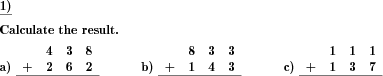Custom math worksheets at your fingertips# Details for problem "Columnar addition"

Quickname: 3290

Elementary School, Primary School, Middle School.

## Example## Description

A series of natural positive numbers have to be added with/ without carry in written columnar form.

This problem providing visual representations of columnar addition will allow young mathematicians to grip columnar addition concepts. It can be used to exercise the columnar addition concept with/ without carries independently, either in the classroom or as a homework assignment. It can also be generated according to the differentiated ability for grades 3 to 5. Boost the addition skills by practicing these worksheets with the following added values.

1. Revise the addition of 2 or more numbers.
2. Learn to add columnar digits with/ without carries.
3. Observe and understand the concept of writing numbers in columns to add.
4. Lay a foundation to use addition skills in future Maths.

These worksheets can be customized for different age groups as per requirement.

• The number of problems can range from 1 to 10.
• The number of summands can be selected from 2 to 4.
• The number of places for the summands can be chosen from 1 to 5.
• Concerning carries, it may be specified that
• no carries may occur
• carries may only occur every other digit so that a column where a carry has been added will never produce another carry
• carries may occur randomly.

The answer key will provide solutions to all problems.

Download free printable worksheets for this math problem here. The worksheet contains the problems only, the solution sheet includes the answers. Just click on the respective link.

•Worksheet 1Solution sheet with answers
•Worksheet 2Solution sheet with answers
•Worksheet 3Solution sheet with answers

If you can not see the solution sheets for download, they may be filtered out by an ad blocker that you may have installed. If this is the case, please allow ads for this page and reload the page. The solution sheets will then reappear.

• Do these sample worksheets do not really fit?
• Do you need more math worksheets, with a different level of difficulty?
• Would you like to combine different problems on a worksheet and adjust them to your needs?
• As a teacher, you can put together your own worksheets using the automatically generated math problems provided.
With a free initial credit, you can start creating your own math worksheets in a few minutes.

You can try it for free! Register here, to create custom worksheets now!

## Customization options for this problem

Parameter
Possible values
Number of problems
1, 2, 3, 4, 5, 6, 7, 8, 9, 10
Number of summands
2, 3, 4
Number of summand digits
1, 2, 3, 4, 5
Carries
never, max every other digit, yes

## Similar problems

Remark
Description
Long addition of numbers with decimals
Columnar addition of multiple decimal fractions.
Similar problem type for subtraction

## dw-Math worksheet templates that do contain this problem

Name
Title
Description